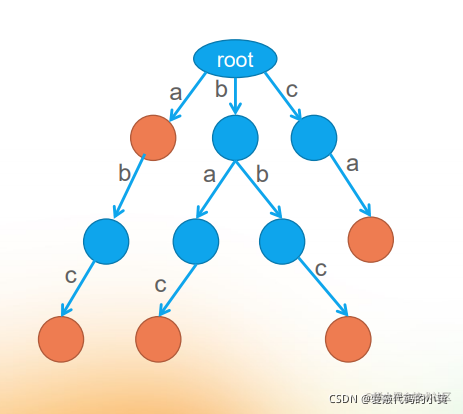# 关键词匹配——HashMap已死，Trie树称王# 二、何为关键词匹配# 三、何为Trie树

• （标橙色的节点是“目标节点“，即根节点到这个目标节点的路径上的所有字母构成了一个单词。）

Trie树功能如下：

• 维护字符串集合（即字典）
• 向字符串集合中插入字符串（即建树）
• 查询字符串集合中是否有某个字符串（即查询）
• 统计字符串在集合中出现的个数（即统计）
• 将字符串集合按字典序排序（即字典序排序）
• 求集合内两个字符串的LCP（Longest CommonPrefix，最长公共前缀）（即求最长公共前缀）

# 四、为什么不直接使用HashMap

String类

``````	// 计算字符串的hashCode
public int hashCode() {
int h = hash;
if (h == 0 && value.length > 0) {
char val[] = value;

for (int i = 0; i < value.length; i++) {
h = 31 * h + val[i];
}
hash = h;
}
return h;
}

HashMap类

``````	public boolean containsKey(Object key) {
return getNode(hash(key), key) != null;
}

static final int hash(Object key) {
int h;
return (key == null) ? 0 : (h = key.hashCode()) ^ (h >>> 16);
}

# 五、Trie树的代码实现## 1、Trie的初始化

• pass：通过该点的字符串数量
• end：结束该点的字符串数量
• tries：子树
``````class Trie {
int pass;
int end;
Trie[] tries;

public Trie() {
pass = 0;
end = 0;
tries = new Trie;
}
}

## 2、Trie添加字符串

``````	public void insert(String word) {
if (word == null) {
return;
}
char[] str = word.toCharArray();
Trie trie = this;
int path = 0;
for (int i = 0; i < str.length; i++) {
path = str[i] - 'a';
// 看一下子树是不是已经存在，如果不存在，则进行创建
if (trie.tries[path] == null) {
trie.tries[path] = new Trie();
}
trie.pass++;
trie = trie.tries[path];
}
trie.end++;
}

## 3、Trie查询字符串出现的频率

``````		/**
* 该字符串出现了几次
*
* @param word
* @return
*/
public int search(String word) {
if (word == null) {
return 0;
}
char[] str = word.toCharArray();
Node node = root;
int path = 0;
for (int i = 0; i < str.length; i++) {
path = str[i] - 'a';
if (node.nodes[path] == null) {
return 0;
}
node = node.nodes[path];
}
return node.end;
}

## 4、Trie删除字符串

• Java选手直接置为 `null` ，GC即可回收，C++选手要手动回收
``````	public void delete(String word) {
if (search(word) == 0) {
return;
}
char[] str = word.toCharArray();
Node node = root;
int path = 0;
for (int i = 0; i < str.length; i++) {
path = str[i] - 'a';
if (--node.nodes[path].pass == 0) {
// Java垃圾回收直接回收掉
node.nodes[path] = null;
return;
}
node = node.nodes[path];
}
node.end--;
}